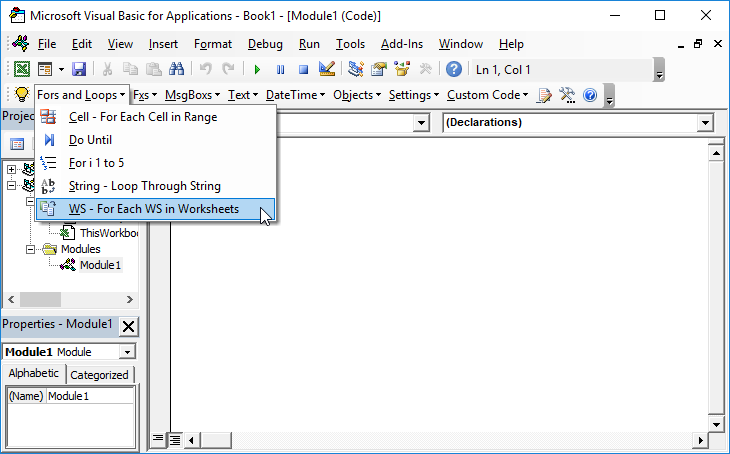# VBA – Autofill with a Macro

## Autofill in VBA

AutoFill is a great tool in Excel when data is repetitive or if it is sequential (like days of the week, dates, months of the year etc.). We would normally fill the first couple of cells with data required, and then use AutoFill to copy the required data down to a selected range.

This can all be done using a VBA macro.

## AutoFill using Incrementing Numbers

Firstly, in our code, we can declare two Range variables.

Selection1 is the range with the data to autofill – for example 1 and 2.

Selection2 is the entire range to autofill, this includes the first range plus the blank spaces to autofill.

We then use the AutoFill method to fill the second selection range.

``````Public Sub MyAutoFill()
'by AutomateExcel.com

'Declare range Variables
Dim selection1 As Range
Dim selection2 As Range

'Set range variables = their respective ranges
Set selection1 = Sheet1.Range("A1:A2")
Set selection2 = Sheet1.Range("A1:A12")

'Autofill
selection1.AutoFill Destination:=selection2

End Sub``````

We can then run the macro to Autofill Range (A1:A12).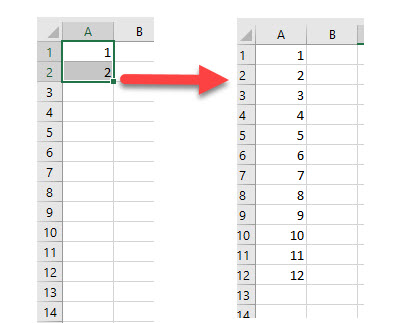When we are using the AutoFill method, we can select the type of Autofill we wish to perform. In the above example, we did not specify the type of Autofill which means the default type was used – in this case, incrementing the numbers by 1 on each line.

## AutoFill using Months

The macro to autofill using months is almost identical to the one used to increment numbers, with one important exception.

``````Public Sub AutoFillMonths()
'by AutomateExcel.com
'Declare range Variables
Dim selection1 As Range
Dim selection2 As Range
'Set range variables = their respective ranges
Set selection1 = Sheet1.Range("A1:A2")
Set selection2 = Sheet1.Range("A1:A12")
'Autofill the months
AutoFill Destination:=selection2, Type:=xlFillMonths
End Sub``````

When we fill in the type of autofill, we are given a number of Excel Constants to choose from.  If we leave this out, Excel will determine the type of data to fill in from the original data given.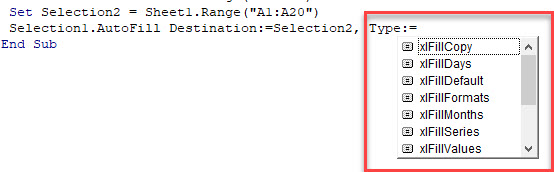Excel is able to pick up standard series like months, days of the week and incremental numbers without the need to use the Type argument however.

## AutoFill using xlFillCopy

If we want to use an AutoFill macro to copy the information down to new cells, we  can use the xlFillCopy Constant.

``````Public Sub AutoFillCopy()
Dim Selection1 As Range
Dim Selection2 As Range
Set Selection1 = Sheet1.Range("A1:A1")
Set Selection2 = Sheet1.Range("A1:A12")
Selection1.AutoFill Destination:=Selection2, Type:=xlFillCopy
End Sub``````

Running this macro would copy the data in Range(“A1”) down to Range(“A1:A12”) rather than auto filling the cells with subsequent months from “Jan”.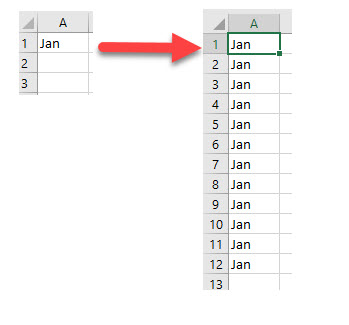## AutoFill using xlFlashFill

When we need to convert text to columns in Excel, we can either use the text to columns option, or we can use an option called Flash Fill.  This is extremely useful in VBA.

Take the following data for example: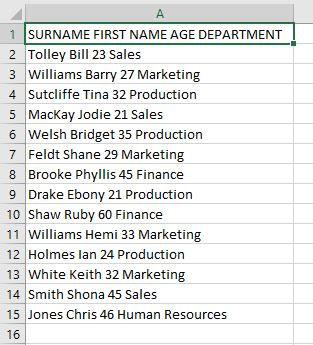We can type the surname “Tolley” in cell B2 and then use a macro to FlashFill the rest of the data.

``````Sub FlashFill()
Dim Selection1 As Range
Dim Selection2 As Range
Set Selection1 = Range("B2:B2")
Set Selection2 = Range("B2:B15")
Selection1.AutoFill Destination:=Selection2, Type:=xlFlashFill
End Sub``````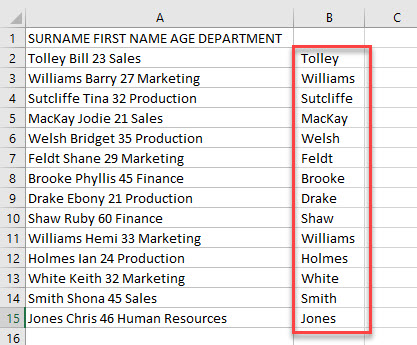We can then repeat this will columns C, D and E to get the remaining data.

`````` Set Selection1 = Range("C2:C2")
Set Selection2 = Range("C2:C15")
Selection1.AutoFill Destination:=Selection2, Type:=xlFlashFill

Set Selection1 = Range("D2:D2")
Set Selection2 = Range("D2:D15")
Selection1.AutoFill Destination:=Selection2, Type:=xlFlashFill

Set Selection1 = Range("E2:E2")
Set Selection2 = Range("E2:E15")
Selection1.AutoFill Destination:=Selection2, Type:=xlFlashFill``````

Each column will then fill with the appropriate data based on the value in row 2.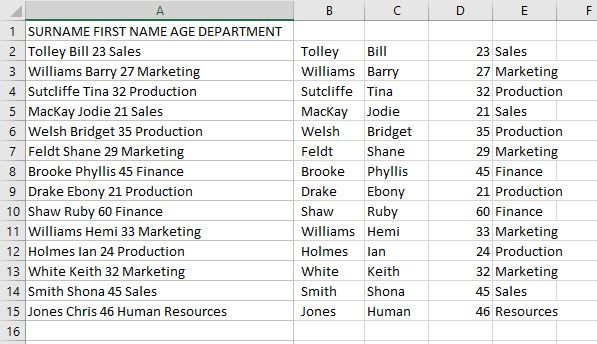Stop searching for VBA code online. Learn more about AutoMacro – A VBA Code Builder that allows beginners to code procedures from scratch with minimal coding knowledge and with many time-saving features for all users!